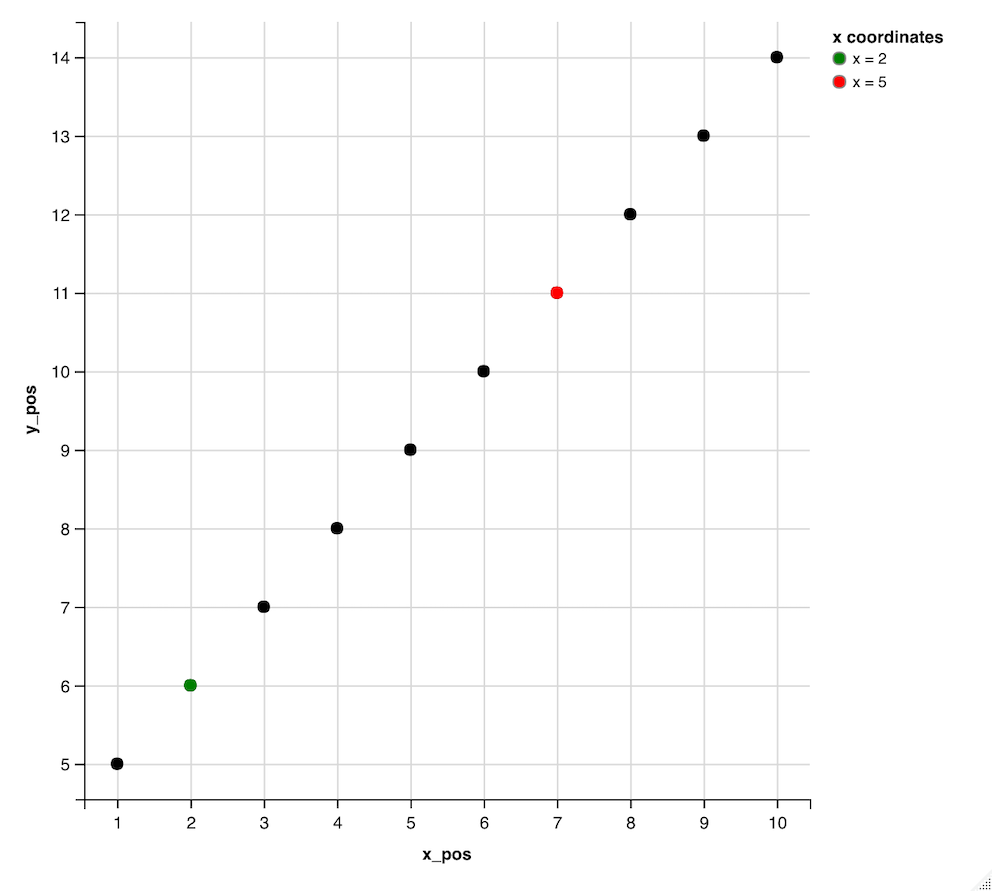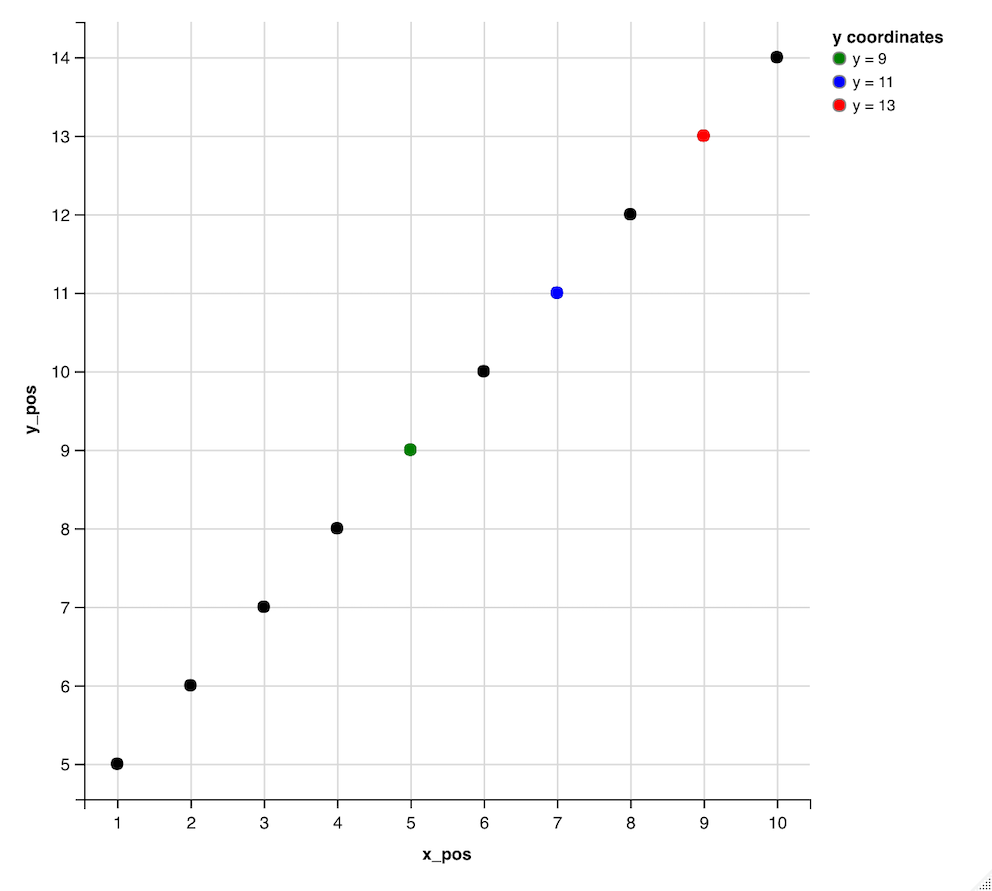# How to add a specific point to legend in ggvis in R?

• Last Updated : 02 Aug, 2022

In this article, we will be going through the approach to add a specific point to a legend in ggvis package in the R programming language.

The ggvis package in R is used to provide the data visualization. It is used to create visual interactive graphics tools for data plotting and representation. The package can be installed into the working space using the following command :

`install.packages("ggvis")`

The ggvis method in the ggvis package is used to start ggvis graphical window. The ggvis method has the following syntax :

ggvis( data , mp1, mp2.,)

Arguments :

• data – The dataset to plot
• mp1, mp2,.. – The map variables to plot

The layer_points() method in the ggvis package is used to mark the coordinates of the points in the form of dots. Visual points can be added to this method, like the fill, stroke, and shape. The layer_points() method has the following syntax in R :

layer_points(vis, data, …)

Arguments :

• vis – The ggvis object
• data – The data frame to be used for plotting the points
• … – The additional attributes like the fill

The scale_nominal() method is used to add a nominal scale to the ggvis plot. The scale_nominal() method is supplied with external visual attributes, like, fill and range. The range attribute is supplied with a vector of colours to label the data points.

`scale_nominal (fill , range )`

This is further supplied with the add_legend method which is used to add a legend to the ggvis plot. The title attribute can be used to add a title to the made plot.

add_legend ( fill , title)

Arguments :

• fill – The visual fill attribute
• title – The title to be given to the legend

Initially, a data frame is created using the specified data points in R. Two subsets of the data frame are created using the subset() methods. The data frame is then subjected to the ggvis method using the pipe operator. The points are then plotted using dots and then the points are represented using the visual fill attribute. The legend is added with a title.

## R

 `# installing the required libraries ``library``(ggplot2)``library``(ggvis)`` ` `# creating the data frame by defining ``# the x and y coordinates respectively``x_pos <- 1:10`` ` `# defining the y axis ``y_pos = 5:14`` ` `# creating the data frame``data_frame = ``data.frame``(x_pos, y_pos )``print``(``"Data Frame"``)``print``(data_frame)``  ` ` ``# creating a subset of the dataset ``# where x_pos value is equivalent to 2``df1 <- ``subset``(data_frame, x_pos == 2)``  ` `# creating a subset of the dataset ``# where x_pos value is equivalent to 2``df2 <- ``subset``(data_frame, x_pos == 7)``  ` `# plotting the tick marks on the axes``data_frame %>%``ggvis``(~x_pos,~y_pos) %>%``   ` `# marking the point labels for ``# the coordinates``layer_points``() %>% `` ` `# marking x = 2 with green color``layer_points``(data = df1, fill = ~``"x = 2"``) %>%`` ` `# marking x = 5 with green color``layer_points``(data = df2, fill = ~``"x = 5"``) %>%``scale_nominal``(``"fill"``, range = ``c``(``"green"``, ``"red"``) ) %>%`` ` `# creating solid color dots``add_legend``(``"fill"``, title = ``"x coordinates"` `)`

Output

``` "Data Frame"
> print(data_frame)
x_pos y_pos
1      1     5
2      2     6
3      3     7
4      4     8
5      5     9
6      6    10
7      7    11
8      8    12
9      9    13
10    10    14```The below code snippet illustrates the plotting of the data frame y coordinates indicated by the y_pos data points. The points are labelled using different colors and fill attribute values respectively.

## R

 `# installing the required libraries``library``(ggplot2)``library``(ggvis)`` ` `# creating the data frame by defining``# the x and y coordinates respectively``x_pos < - 1: 10`` ` `# defining the y axis``y_pos = 5: 14`` ` `# creating the data frame``data_frame = ``data.frame``(x_pos, y_pos)``print``(``"Data Frame"``)``print``(data_frame)`` ` `# creating a subset of the dataset where``# y_pos value is equivalent to 9``df1 < - ``subset``(data_frame, y_pos == 9)`` ` `# creating a subset of the dataset where``# y_pos value is equivalent to 11``df2 < - ``subset``(data_frame, y_pos == 11)`` ` `# creating a subset of the dataset where``# y_pos value is equivalent to 13``df3 < - ``subset``(data_frame, y_pos == 13)`` ` `# plotting the tick marks on the axes``data_frame % >%``ggvis``(~x_pos, ~y_pos) % >%`` ` `# marking the point labels for the``# coordinates``layer_points``() % >%`` ` `# marking x = 2 with green color``layer_points``(data=df1, fill=~``"y = 9"``) % >%``layer_points``(data=df2, fill=~``"y = 11"``) % >%``layer_points``(data=df3, fill=~``"y = 13"``) % >%``scale_nominal``(``"fill"``, range=``c``(``"green"``, ``"blue"``, ``"red"``)) % >%`` ` `# creating solid color dots``add_legend``(``"fill"``, title=``"y coordinates"``)`

Output

``` "Data Frame"
> print(data_frame)
x_pos y_pos
1      1     5
2      2     6
3      3     7
4      4     8
5      5     9
6      6    10
7      7    11
8      8    12
9      9    13
10    10    14```My Personal Notes arrow_drop_up